﻿ Illumuitar## Signal Processing

• My group of 3 built an Audio Spectrum visualiser and guitar tuner and displayed them through a custom LED matrix on the face of a guitar.
• I designed, assembled and programmed a custom PCB and used time and frequency domain signal processing.

## How it Works

The guitar has two main functions; The spectrum visualiser works by applying a Fast Hartley Transform algorithm to a microphone input and splitting the detected frequencies logarithmically into 8 bins. These are then scaled and displayed on the LED matrix through a look up table.

```maparray = map(fht_oct_out,35,120,0,15);
for (int m=0; m <=6; m++){ //iterate through frequency bins
for(int j=(26-(4*m)); j >= (26-(4*(m+1))); j--){ //iterate across matrix
for(int i=0; i <= maparray[m]; i++){
if ((lookuptable[i][j] != 0) && (i < 16) && (j >=0)) { //find corresponding value in looKup table
strip.setPixelColor(lookuptable[i][j]-1, r,g,b); //set colour
}
for(byte k = 15; k > maparray[m]; --k){ //turn all the other LEDs off
if ((lookuptable[k][j] != 0) && (j >=0)){
strip.setPixelColor(lookuptable[k][j]-1, 0,0,0);```

The guitar tuner takes the analog output from the guitar's pickups and amplifies the signal to 5V. A DC offset of 2.5V is applied to keep the signal positive. This signal can then be read by the 10-bit ADC of the Atmega MCU and an autocorrelation method is used to detect precise frequencies and compare them to the known correct values.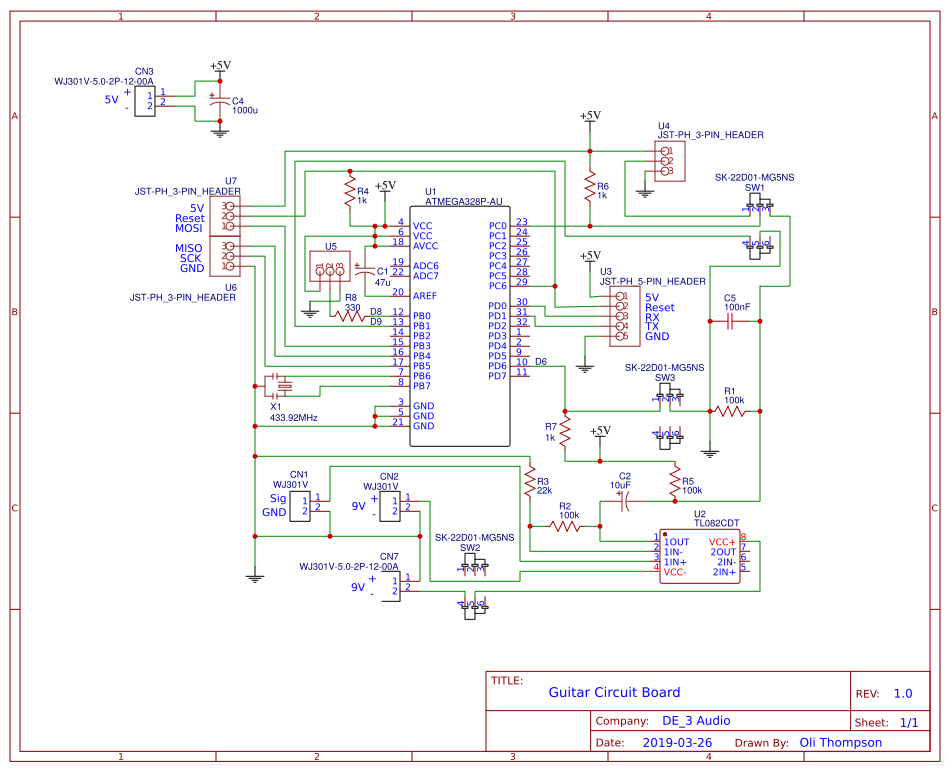### Circuit Schematic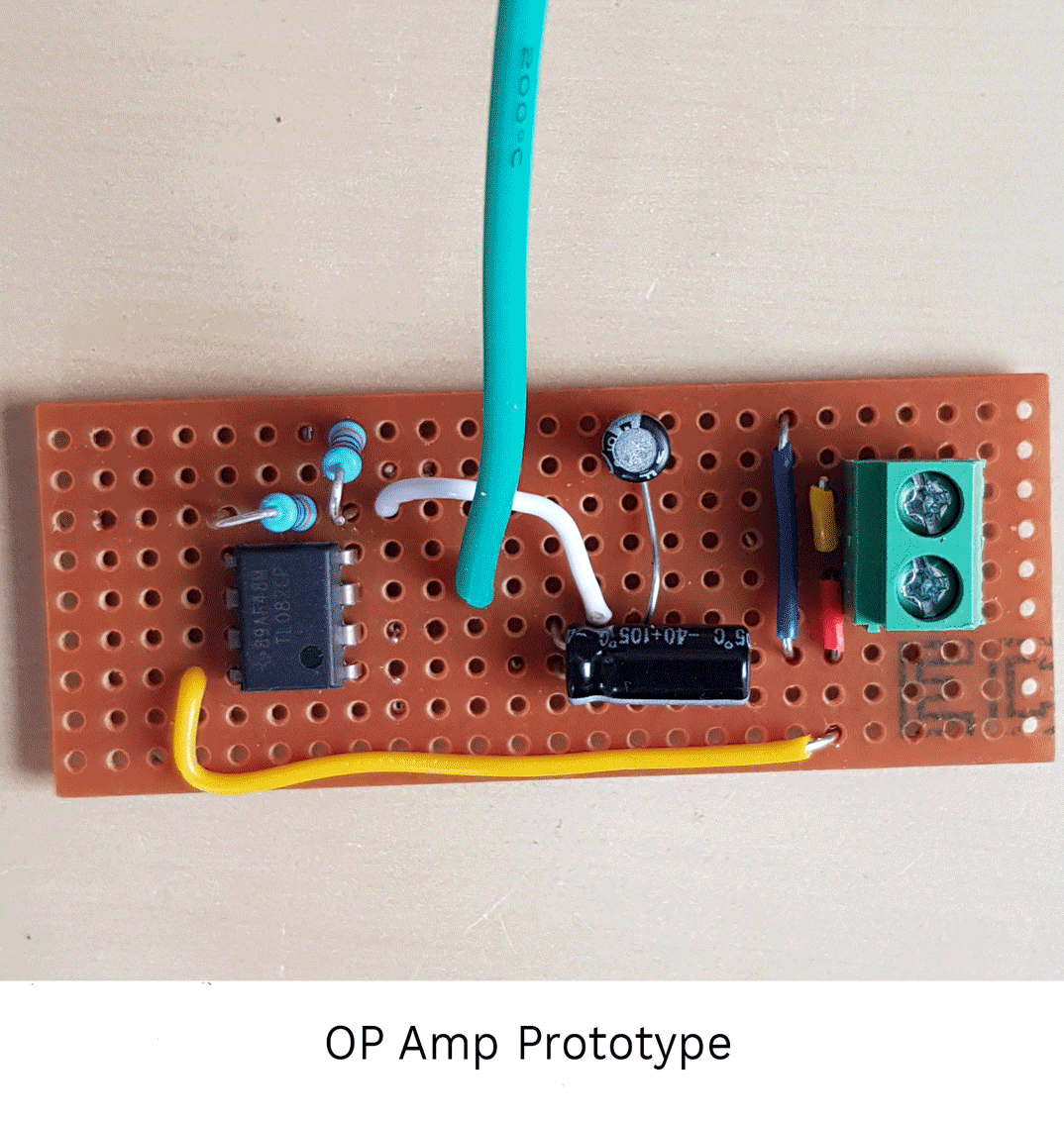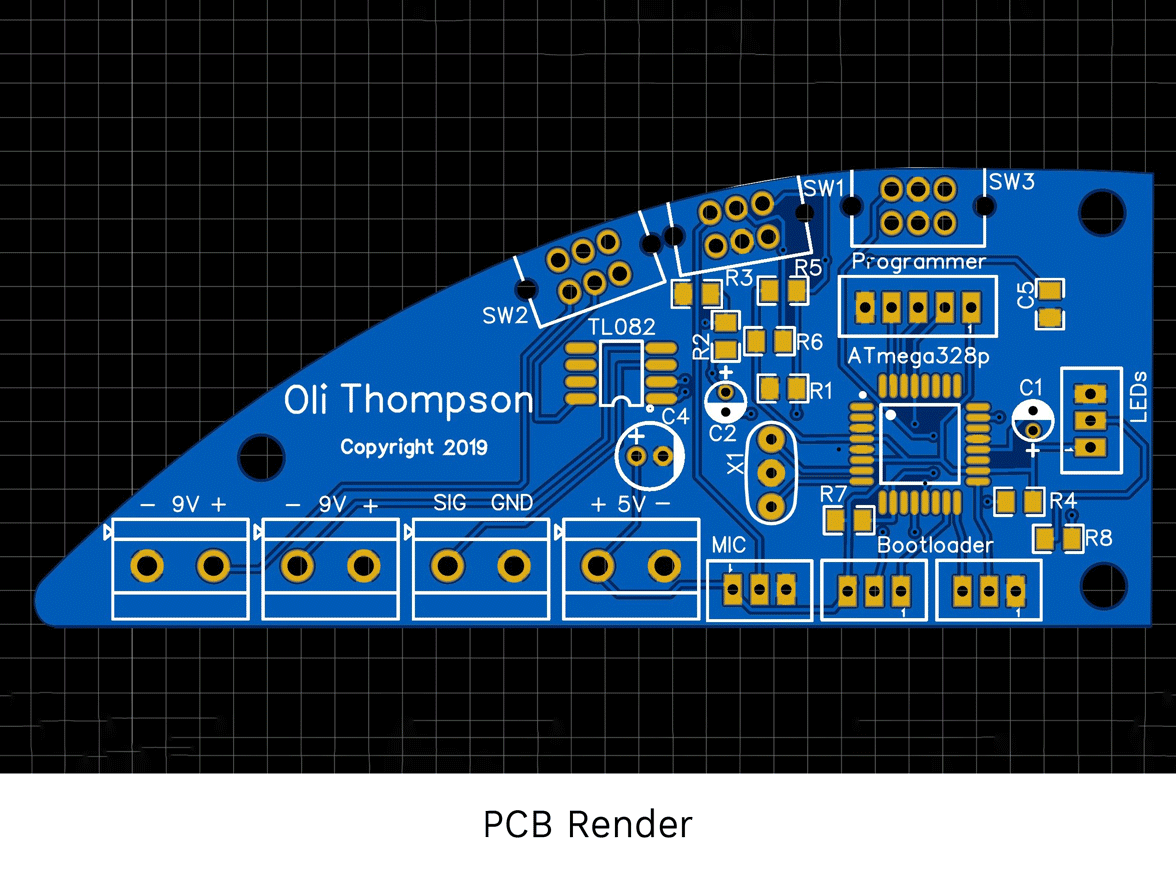## My Contribution

• I generated the circuit schematics
• I designed and populated the custom PCB
• I programmed the look-up table and the spectrum visualiser in C++
• I was responsible for a large part of the mechanical design and manufacture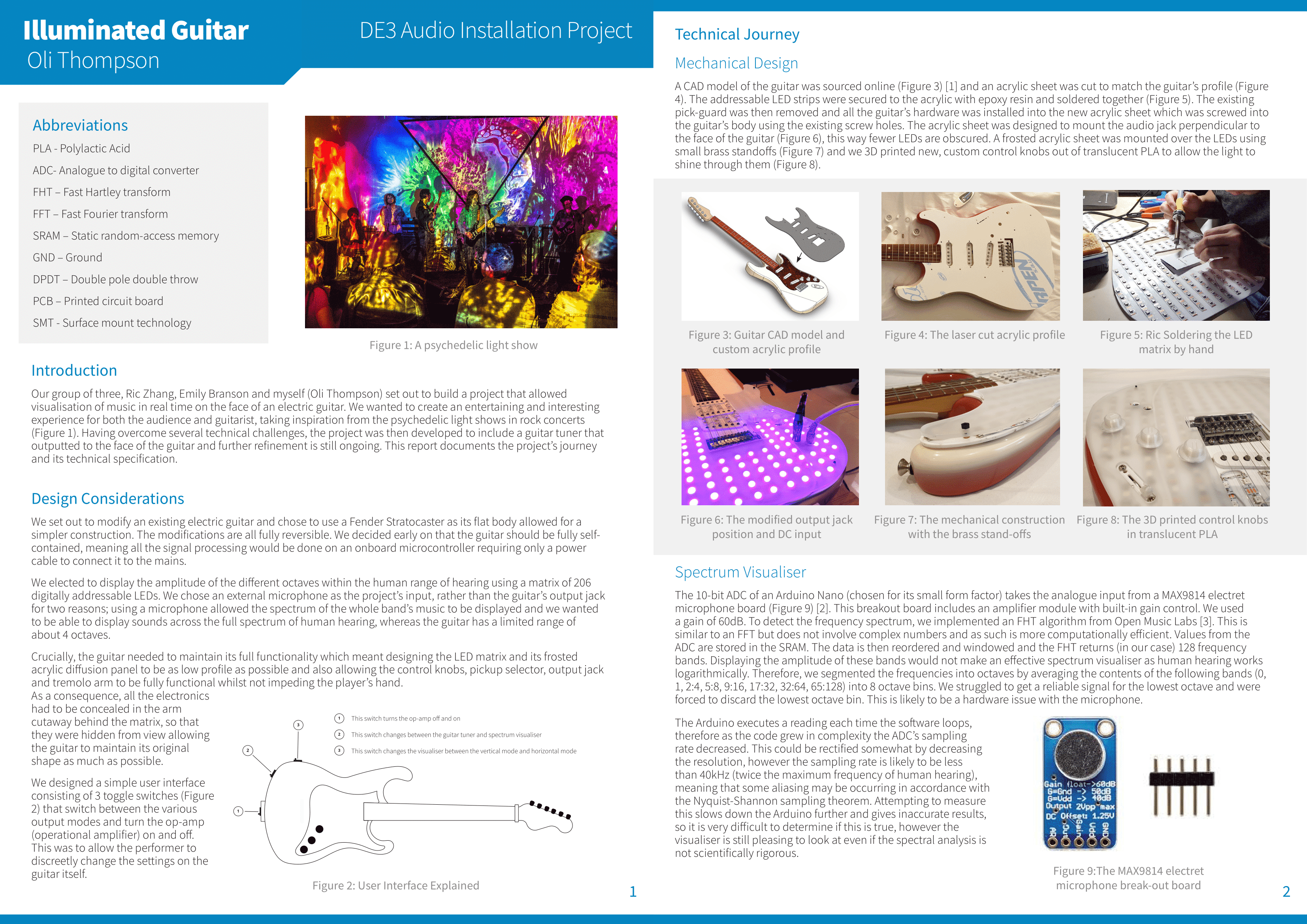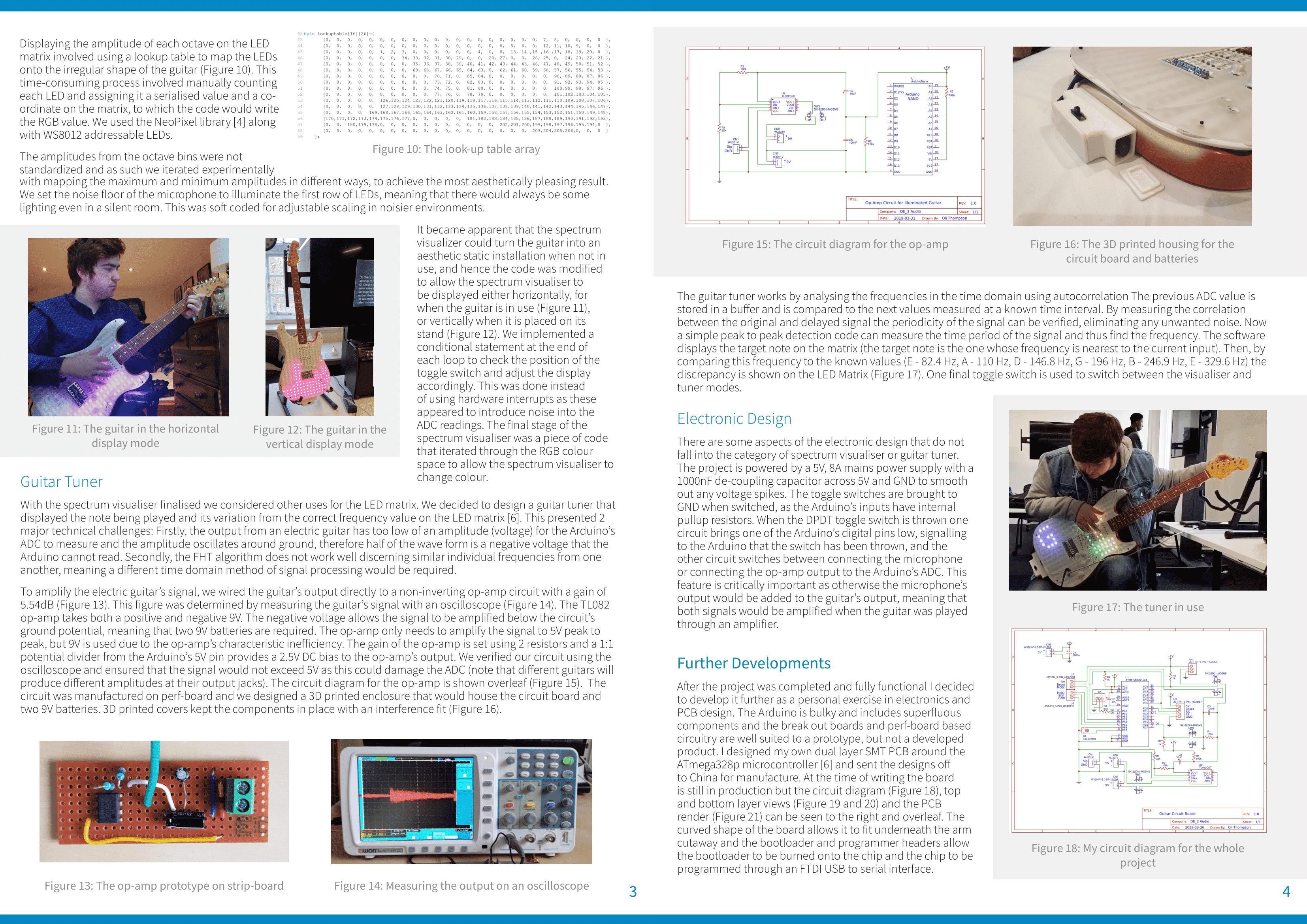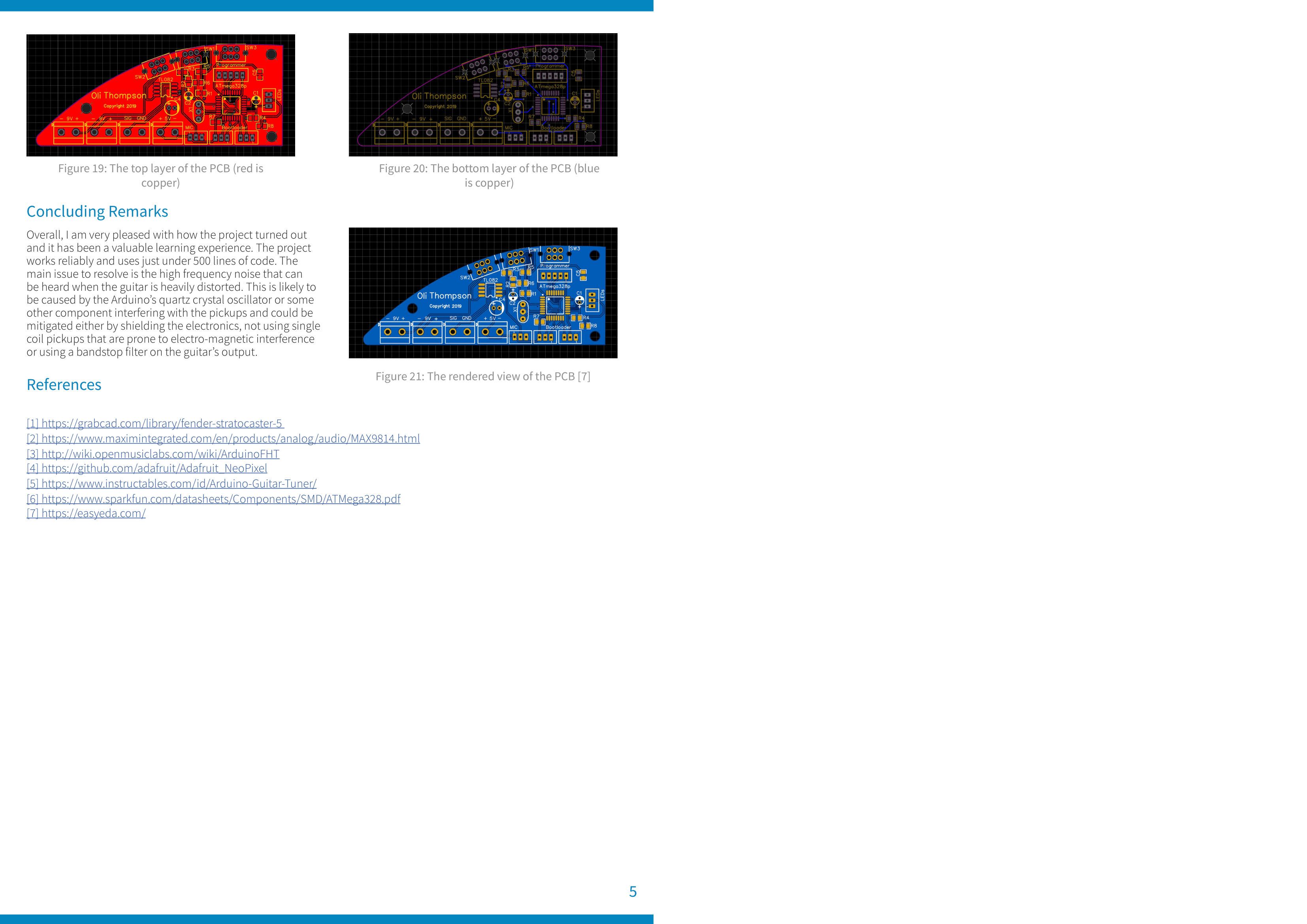<